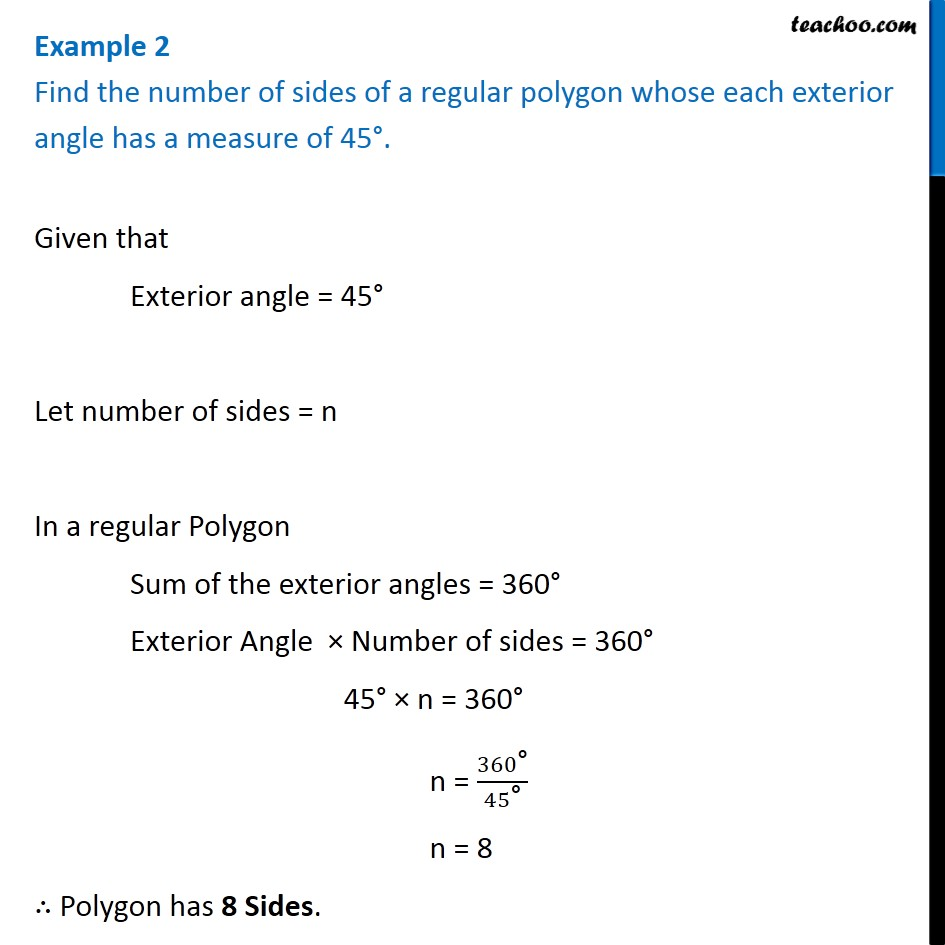Examples

Chapter 3 Class 8 Understanding Quadrilaterals
Serial order wiseGet live Maths 1-on-1 Classs - Class 6 to 12

### Transcript

Example 2 Find the number of sides of a regular polygon whose each exterior angle has a measure of 45°. Given that Exterior angle = 45° Let number of sides = n In a regular Polygon Sum of the exterior angles = 360° Exterior Angle × Number of sides = 360° 45° × n = 360° n = 360"°" /45"°" n = 8 ∴ Polygon has 8 Sides.